# Chemistry Behavior of Gases Review

On a piece of paper, write the answers that would fill in the blanks. After you have completed that, you can highlight the paragraph to reveal the answers.

John         Dalton was the first to form a hypothesis about partial    pressure of combined gases. After experimenting with gases, he concluded that each gas exerts the same pressure it would if it      alone were present at the same temperature. If a gas is collected over water, the pressure in the container actually includes the sum of the vapor      pressure of the gas and the       water vapor pressure. Consequently, we must       subtract the water vapor pressure from the total pressure to obtain the pressure of the          gas alone.
Avogadro’s law states that equal volumes of different gases, at the same                    temperature and                pressure, contain the same             number of                       molecules.

Word Bank:

gas                              pressure (used 3 times)           subtract            molecules                          Dalton                        temperature                               water            number           alone

Using the formulas that you learned, work the following problems. Determining which gas law problem to use is the key. Do this by labeling the data given, identifying the data that the problem is looking for, and matching those things to the different gas laws. Refer to your gas laws formula sheet as a reference.

To check the solutions to the problems, click on Step 1, Step 2, and then Step 3 for explanations.

1. A 952 cm3 container of gas is exerting a pressure of 108 kPa while at a temperature of 48 °C. Calculate the pressure of this same amount of gas in a 1236 cm3 container at a temperature of 64 °C.

Step 1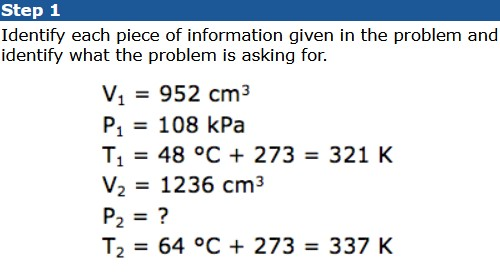Step 2Step 3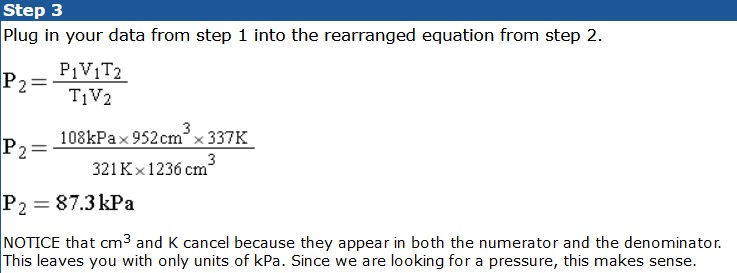2. At STP, a sample of gas occupies 24.5 mL. Calculate the volume of this gas at a pressure of 2.3 atm and a temperature of 301 K.

Step 1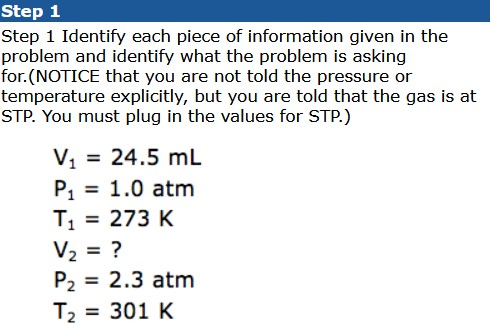Step 2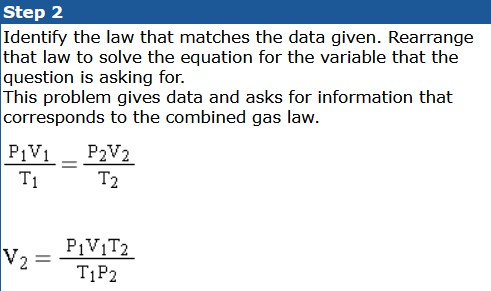Step 3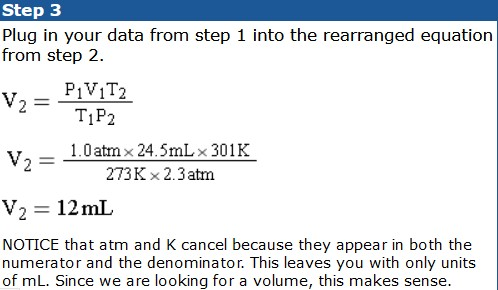3. A 3.25 L container of ammonia gas exerts a pressure of 652 mm Hg at a temperature of 243 K. Calculate the pressure of this same amount of gas in a 2.50 L container at a temperature of 311 K.

Step 1Step 2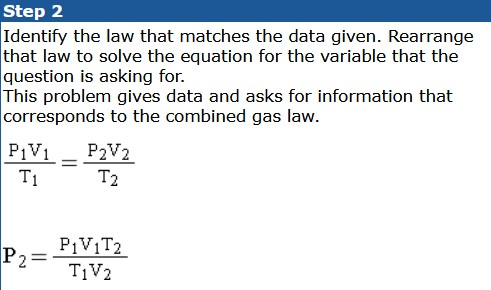Step 34. A sample of gas has a volume of 5.23 cm3 at a pressure of 72.6 kPa and a temperature of 25 °C. What will be the volume of the gas if the pressure is changed to 124 kPa and the temperature is changed to 0 °C?

Step 1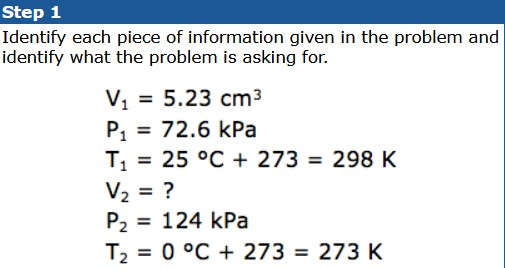Step 2Step 35. Calculate the pressure (in kPa) of 0.421 mole of helium gas at 254 K when it occupies a volume of 3.32 L.

Step 1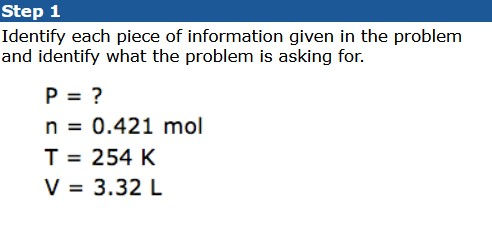Step 2Step 36. How many moles of argon are there in a 22.4 L sample of gas at 101.3 kPa and 0 °C?

Step 1Step 2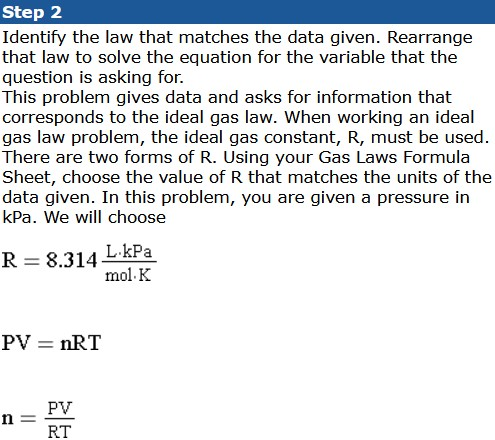Step 37. What is the volume of 2.56 moles of gas at 0.634 atm and 65 °C?

Step 1Step 2Step 3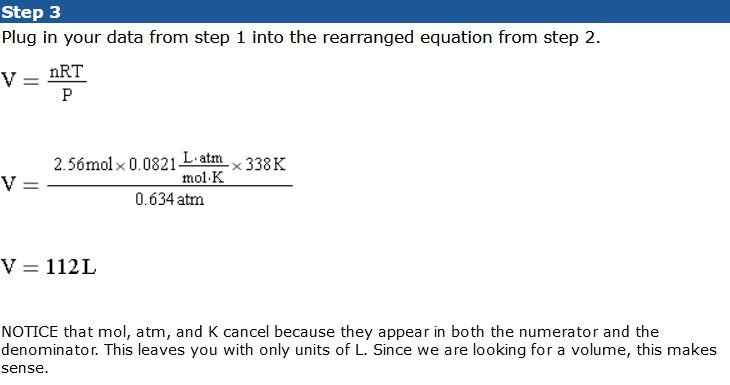8. A 500.0 g block of dry ice (solid CO2, molar mass = 44.0 g) vaporizes to a gas at room temperature. Calculate the volume of gas produced at 25.0 °C and 1.50 atm.

Step 1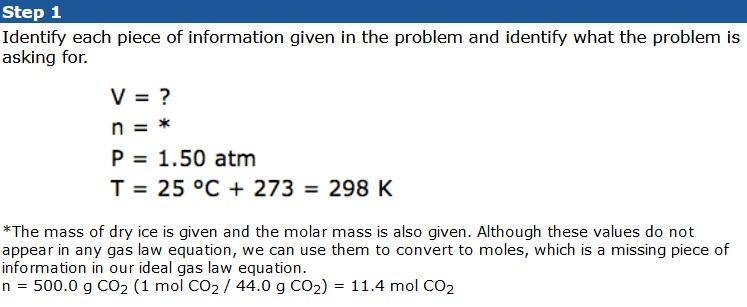Step 2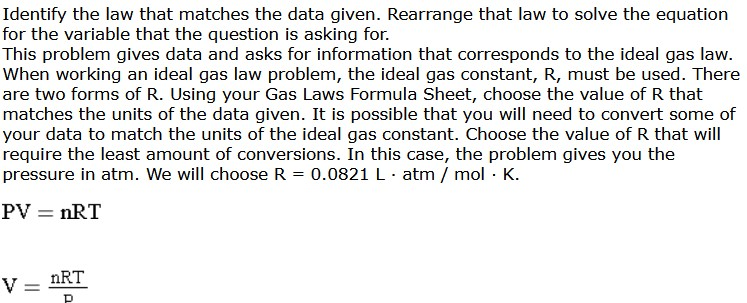Step 3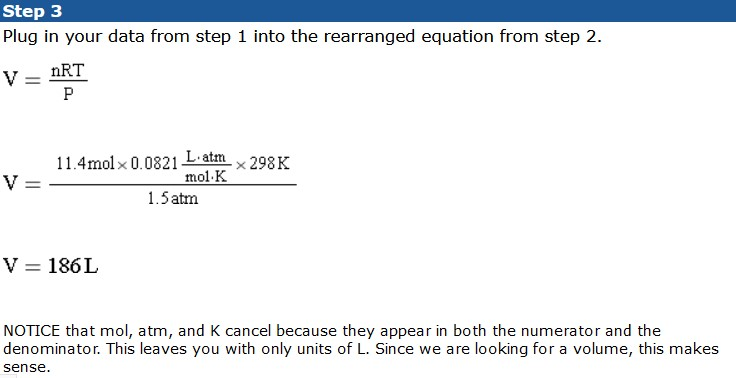source